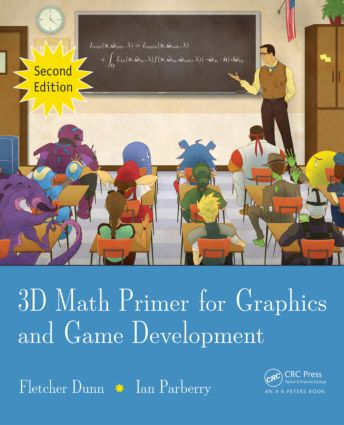# 3D Math Primer for Graphics and Game Development

## 2nd Edition

A K Peters/CRC Press

846 pages

Hardback: 9781568817231
pub: 2011-11-02
\$89.95
x
eBook (VitalSource) : 9781498759892
pub: 2015-09-15
from \$28.98

FREE Standard Shipping!

### Description

This engaging book presents the essential mathematics needed to describe, simulate, and render a 3D world. Reflecting both academic and in-the-trenches practical experience, the authors teach you how to describe objects and their positions, orientations, and trajectories in 3D using mathematics. The text provides an introduction to mathematics for game designers, including the fundamentals of coordinate spaces, vectors, and matrices. It also covers orientation in three dimensions, calculus and dynamics, graphics, and parametric curves.

### Reviews

"With solid theory and references, along with practical advice borne from decades of experience, all presented in an informal and demystifying style, Dunn & Parberry provide an accessible and useful approach to the key mathematical operations needed in 3D computer graphics."

—Eric Haines, author of Real-Time Rendering

"The book describes the mathematics involved in game development in a very clear and easy to understand way, layered on the practical background of years of game engine programming experience."

—Wolfgang Engel, editor of GPU Pro

Cartesian Coordinate Systems

1D Mathematics

2D Cartesian Space

3D Cartesian Space

Odds and ends

Vectors

Vector — mathematical definition and other boring stuff

Vector — a geometric definition

Specifying vectors using Cartesian coordinates

Vectors vs. points

Negating a vector

Vector multiplication by a scalar

Vector magnitude (length)

Unit vectors

The distance formula

Vector dot product

Vector cross product

Linear algebra identities

Multiple Coordinate Spaces

Why multiple coordinate spaces?

Some useful coordinate spaces

Coordinate space transformations

Nested coordinate spaces

In defense of upright space

Introduction to Matrices

Matrix — a mathematical definition

Matrix — a geometric interpretation

The bigger picture of linear algebra

Matrices and Linear Transformations

Rotation

Scale

Orthographic projection

Reection

Shearing

Combining transformations

Classes of transformations

More on Matrices

Determinant of a matrix

Inverse of a matrix

Orthogonal matrices

4 x 4 homogeneous matrices

4 x 4 matrices and perspective projection

Polar Coordinate Systems

2D Polar Space

Why would anybody use Polar coordinates?

3D Polar Space

Using polar coordinates to specify vectors

Rotation in Three Dimensions

What exactly is "orientation?"

Matrix form

Euler angles

Axis-angle and exponential map representations

Quaternions

Comparison of methods

Converting between representations

Geometric Primitives

Representation techniques

Lines and rays

Spheres and circles

Bounding boxes

Planes

Triangles

Polygons

Mathematical Topics from 3D Graphics

How graphics works

Viewing in 3D

Coordinate spaces

Polygon meshes

Texture mapping

The standard local lighting model

Light sources

Skeletal animation

Bump mapping

The real-time graphics pipeline

Some HLSL examples

Mechanics 1: Linear Kinematics and Calculus

Overview and other expectation-reducing remarks

Basic quantities and units

Average velocity

Instantaneous velocity and the derivative

Acceleration

Motion under constant acceleration

Acceleration and the integral

Uniform circular motion

Mechanics 2: Linear and Rotational Dynamics

Newton's three laws

Some simple force laws

Momentum

Impulsive forces and collisions

Rotational dynamics

Real-time rigid body simulators

Curves in 3D

Parametric polynomial curves

Polynomial interpolation

Hermite curves

Bezier curves

Subdivision

Splines

Hermite and Bezier splines

Continuity

Automatic tangent control

Afterword

What next?

Appendix A: Geometric Tests

Appendix B: Answers to the Exercises

Bibliography

Index

Exercises appear at the end of each chapter.

Fletcher Dunn has been programming video games professionally since 1996. He served as principle programmer at Terminal Reality in Dallas, where he was one of the architects of the Infernal engine and lead programmer on BloodRayne. He was a technical director for the Walt Disney Company at Wideload Games in Chicago, where he was the lead programmer for Disney Guilty Party, which won IGN's Family Game of the Year at E3 2010. He is currently a developer at Valve Software in Bellevue, Washington.

Ian Parberry is a professor in the Department of Computer Science and Engineering at the University of North Texas. Dr. Parberry has more than a quarter century of experience in research and teaching and is nationally known as one of the pioneers of game programming in higher education.